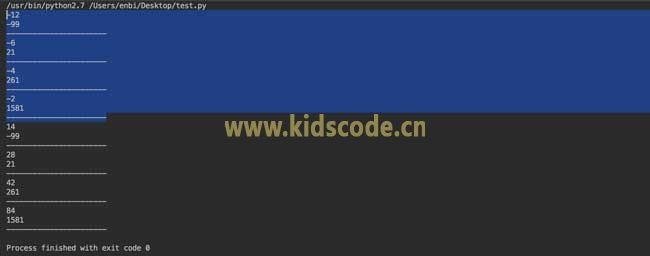1、则：x + 100 = n2, x + 100 + 168 = m2

2、计算等式：m2 - n2 = (m + n)(m - n) = 168

3、假设： m + n = i，m - n = j，那么i * j =168，168是偶数，可推断i 和 j 至少一个是偶数

4、再由上一步的算式可得： m = (i + j) / 2， n = (i - j) / 2，因为m、n都是整数，所以i+j与i-j都是偶数，则i 和 j 要么都是偶数，要么都是奇数。

5、从 3 和 4 推导可知道，i 与 j 均是偶数。

6、由于 i * j = 168，i与j同正或者同负，且不等于0， j>=2，则 1 < i < 168 / 2 + 1 或 j<=-2，则 -1 > i > 168 / -2 - 1。

7、接下来将 i 的所有数字循环计算即可。

#!/usr/bin/python

# -*- coding: UTF-8 -*-

for i in range(-85, 85):

if i == 0:

continue

if 168 % i == 0:

j = 168 / i

if i > j and (i + j) % 2 == 0 and (i - j) % 2 == 0:

print (i)

m = (i + j) / 2

n = (i - j) / 2

x = n * n - 100

print (x)

print ('-----------------------')